Click to Chat

1800-1023-196

+91-120-4616500

CART 0

• 0

MY CART (5)

Use Coupon: CART20 and get 20% off on all online Study Material

ITEM
DETAILS
MRP
DISCOUNT
FINAL PRICE
Total Price: Rs.

There are no items in this cart.
Continue Shopping• Complete Physics Course - Class 11
• OFFERED PRICE: Rs. 2,968
• View Details

Graphical Representation and Equations of Motion

Table of Content

Position - Time Graph

The Velocity - Time Graph

Problem 3 (JEE Main)

Related Resources

While studying motion of bodies we have to keep two things in mind. We have to study various characteristics associated with moving bodies while we are to explore and study in detail, about the cause of producing motion. Kinematics is the branch of physics which deals only with the description of motion of bodies. Mainly there are two type of graphs are belonging to the kinematics. One is  position-time graph and the other is velocity-time graph.

These graphs will have validity only if motion under study is along a straight line. Then, displacement, velocity and acceleration vectors are collinear and can be treated as algebraic quantities. Let x-axis be the path of motion. Then, x-coordinate represents the magnitude of position vector.

Position - Time GraphIf we plot time t along the x-axis and the corresponding position (say x) from the origin O on the y-axis, we get a graph which is called the position-time graph. This graph is very convenient to analyse different aspects of motion of a particle. Let us consider the following cases.

(i) In this case, position (x) remains constant but time changes. This indicates that the particle is stationary in the given reference frame. Hence, the straight line nature of position-time graph parallel to the time axis represents the state of rest. Note that its slope (tan θ) is zero.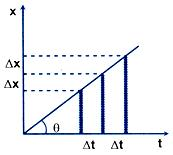(ii) When the x-t graph is a straight line inclined at some angle (θ≠) with the time axis, the particle traverses equal displacement Δx in equal intervals of time Δt. The motion of the particle is said to be uniform rectilinear motion. The slope of the line measured by Δx/Δt = tθ represents the uniform velocity of the particle.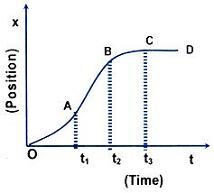(iii)    When the x-t graph is a curve, motion is not uniform. It either speeds up or slows down depending upon whether the slope (tan θ successively increases or decreases with time. As shown in the figure the motion speeds up from t = 0 to t=t1 (since the slope tan θ increases). From t=t1 to t=t2, AB represents a straight line indicating uniform motion. From t=t2 to t=t3, the motion slows down and for t>t3 the particle remains at rest in the reference frame.

Problem 1:-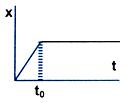The adjacent figure shows the displacement-time graph of a particle moving on the x-axis. Choose the correct option given below.

(A)      The particle is continuously going in positive x direction.

(B)      The particle is at rest.

(C)      The particle moves at a constant velocity upto a time t0, and then stops.

(D)      The particle moves at a constant acceleration upto a time t0,and then stops.

Solution:

The correct option is (C). Up to time t0, particle is said to have uniform rectilinear motion and after that comes to rest as the slope is zero.

The Velocity - Time Graph

The velocity-time graph gives three types of information.(i) The instantaneous velocity.

(ii) The slope of the tangent to the curve at any point gives instantaneous acceleration.

a = dv/dt = tan θ

(iii)    The area under the curve gives total displacement of the particle.

Now, let us consider the uniform acceleration. The velocity-time graph will be a straight line.The acceleration of the object is the slope of the line CD.

a = tan θ = BC/BD = (v-u)/t

v = u +at        …... (1)

The total displacement of the object is area OABCD

s = Area OABCD = Area of rectangle OABD + Area of triangle BCD

s = ut + (1/2)at2          …... (2)

Again, s = Area OABCD

= 1/2(AC + OD) x OA = 1/2(v + u)xt

Problem 2:-From the velocity-time plot shown in figure, find

(a)distance travelled by the particle during the first 40 seconds.

(b)displacement travelled by the particle during the first 40  seconds.

(c) Also find the average velocity during the period.

Solution:(a) Distance = area under the curve = 1/2 x 20 x 5 + 1/2 x 5 x 20

For distance measurement, the curve is plotted as in Fig. (a)

(b) Displacement = area under the curve in Fig. (b) = 0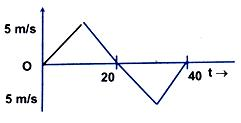(c) Vav = Displacement/time

As displacement is zero,

Thus, Vav = 0

Therefore average velocity during the period will be zero.

Problem 3 (JEE Main)

Two cars A and B cross a point P with velocities 10 m/s and 15 m/s. After that they move with different uniform accelerations and the car A overtakes B with a speed of 25 m/s. Find out the velocity of B at that instant?

Solution:

Let aA = a1 and aB = a2

(25)2 = (10)2+2a1S

Or, 2a1S = 525                      ….... (1)

25 = 10+a1t

So, a1t = 15                         …... (2)

Let velocity of B at that instant is v. Then

v2 = (15)2+2a2S

Or, 2a2S = v2-225               …... (3)

v = 15+a2t

So, a2t = v – 15                  …... (4)

From equations (1) and (2),

2S/t  = 525/15 = 35

and from equations (3) and (4),

2S/t = v2-225/v-15

So, 35 = v2-225/v-15

Solving these two equations we get,

v = 20 m/s

Therefore from the above observation we conclude that, the velocity of B at that instant would be 20 m/s.

Problem 4:-

The velocity-time graph of a moving object is given in the figure. Find the maximum acceleration of the body and distance travelled by the body in the interval of time in which this acceleration exists.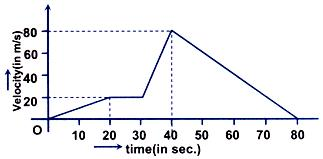Solution:

Acceleration is maximum when slope is maximum,

amax  = (80-20)/(40-30) = 5m/s2

S = 20 m/s x 10 s + 1/2 x 6 m/s2 x 100s2 = 500m

Problem 5:-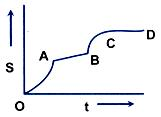The displacement versus time curve is given:

Column I                                    Column II

(a)    OA                             (p)    Velocity increase with time linearly

(b)    AB                             (q)    Velocity decreases with time

(c)    BC                              (r)     Velocity is independent of time

(d)    CD                             (s)    Velocity is zero

Solution:

(a) → (p)      (b)  → (r)           (c)  → (q)          (d) → (s)

This is so because slope of displacement-time curve gives instantaneous velocity.

Refer this video for better understanding about equation of motion:-

The acceleration-time graph:-

Acceleration time curves give information about the variation of acceleration with time. Area under the acceleration time curve gives the change in velocity of the particle in the given time interval.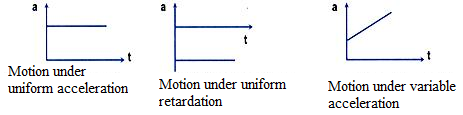Refer this simulation that shows a car traveling along a graph:-

The purpose is to show that, the car can traverse the graph, then it's first derivative is a continuous function.The displacement can have positive, negative or zero value.

The displacement is never greater than the actual distance travelled.

A change in either speed or direction of motion results in a change in velocity.

It is not possible for a particle to possess zero speed with a non-zero velocity.

The velocity-time graph for a uniformly accelerated body is a straight line.Question 1:-

The position-time graph of a body is parallel to t-axis. The body is said to be moving with:

(a) uniform velocity                                              (b) non-uniform velocity

(c) may be uniform or npn-uniform velocity             (d) no motion at all

Question 2:-

The relation between time t and distance x is t = ax2+bx, where a and b are constants. The acceleration is:

(a) 2bV3              (b) -2abV2

(c) 2aV2             (d) -2aV3

Question 3:-

A car has to cover the distance 60 km, if half of the total time it travels with velocity 80 km/h and in rest half time its speed becomes 40 km/h, the average speed of car will be,

(a) 60 km/h         (b) 80 km/h

(c) 120 km/h       (d) 180 km/h

Question 4:-

In a straight line motion the distance travelled is propertional to the square root of the time taken. The acceleration of the particle is proportional to:

(a) velocity            (b) V2

(c) V3                   (d) √V

Question 5:-

The displacement of a particle along a straight line at time t is given by x = b0+b1t+b2t2. The acceleration of the particle is:

(a) b0                (b) b1

(c) 2b2              (d) b2/2Q.1
Q.2
Q.3
Q.4
Q.5

d

d

a

c

c

Related Resources

You might like to refer Kinematics.

For getting an idea of the type of questions asked, refer the  Previous Year Question Papers.

To read more, Buy study materials of Kinematics comprising study notes, revision notes, video lectures, previous year solved questions etc. Also browse for more study materials on Physics here.### Course Features

• 101 Video Lectures
• Revision Notes
• Previous Year Papers
• Mind Map
• Study Planner
• NCERT Solutions
• Discussion Forum
• Test paper with Video Solution